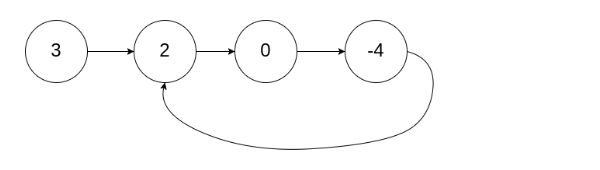11

Detect the first node of the loop

Difficulty: MEDIUM
Avg. time to solve
10 min
Success Rate
90%

Problem Statement

A cycle occurs when a node's next pointer points back to a previous node in the list. The linked list is no longer linear with a beginning and end—instead, it cycles through a loop of nodes.

Input Format :
The first line contains an integer ‘T’ denoting the number of test cases. Then each test case follows.

The first line of each test case contains the elements of the singly linked list separated by a single space and terminated by -1 and hence -1 would never be a list element.

The second line contains the integer position "pos" which represents the position (0-indexed) in the linked list where the tail connects to. If "pos" is -1, then there is no cycle in the linked list.
Output Format :
For each test case, print the integer position “pos” which represents the position of (0-indexed) in the linked list which is the first node of the cycle. Print -1 if there is no cycle in the linked list.

Print the output of each test case in a separate line.
Note:
You are not required to print the expected output; it has already been taken care of. Just implement the function.
Constraints :
0 <= T <= 50
-10^4 <= N <= 10^4
-1 <= pos < N
-10^9 <= data <= 10^9 and data != -1

Time Limit: 1 sec
Can you do this in O(N) time and usingconstant space?
2
3 2 0 -4 -1
1
1 -1
1
1
0
Explanation For Sample Input 1 :
For the first test case,For the second test case, the cycle starting from the first node exists in the linked list.
2
1 2 -1
1
1 2 3 -1
-1
Sample Output 2 :
0
-1Console